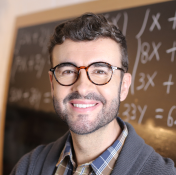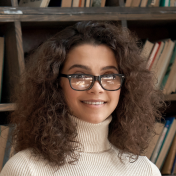# Trust the nation's largest network for Multivariable Calculus tutors

## Find a great match with ourGood Fit Guarantee

#### Featured by the nation’s most respected news sources#### Tutors from top universitiesGet 1:1 help fast

# The Best Multivariable Calculus Tutoring Online

## Find the best Multivariable Calculus tutorLarissa D.
60/hour

Effective Calculus Tutor with over 7 years of tutoring experience across Calculus AB, BC and Multivariable calculus, Business Calculus, Differential Equations, Linear Algebra, Precalculus, Algebra I and II, Trigonometry, Geometry. I am a... See Larissa's full profile

4.9 (37)
60/hour
139 hours tutoring
Response time: 16 minutes
Note that average response times may vary depending on a variety of factors, including time of day.

Effective Calculus Tutor with over 7 years of tutoring experience across Calculus AB, BC and Multivariable calculus, Business Calculus, Differential Equations, Linear Algebra, Precalculus, Algebra I and II, Trigonometry, Geometry. I am a...See Larissa's full profilePromise C.
96/hour

### Experienced College Math Tutor: MultivariableCalculus, Algebra, Trig

I currently tutor all levels of Algebra, Geometry, Trigonometry, Pre-Calculus, Calculus (high school; I and II; AP AB, and AP BC), and Multivariable Calculus (i.e. Calculus 3). I also occasionally tutor Ordinary Differential Equations,... See Promise's full profile

5.0 (1083)
96/hour
3,288 hours tutoring

I currently tutor all levels of Algebra, Geometry, Trigonometry, Pre-Calculus, Calculus (high school; I and II; AP AB, and AP BC), and Multivariable Calculus (i.e. Calculus 3). I also occasionally tutor Ordinary Differential Equations,...See Promise's full profileJosh G.
75/hour

### Caring, Patient, and Versatile Calculus Tutor With 6+ Years Experience

I tutor both Single Variable and Multivariable Calculus. I have a proven record of helping over 100 Calculus students improve their grades to an A, as well as helping my AP Calculus students score a 5 on their AP exams. In addition to my... See Josh's full profile

5.0 (463)
75/hour
1,624 hours tutoring

I tutor both Single Variable and Multivariable Calculus. I have a proven record of helping over 100 Calculus students improve their grades to an A, as well as helping my AP Calculus students score a 5 on their AP exams. In addition to my...See Josh's full profileLisa O.
140/hour

### Caltech Grad, Experienced Math Tutor: AP Calc AB, BC & Calc 1,2,3

I enjoy helping students reach their academic goals in high school and college math courses such as algebra 2, trigonometry, precalculus, calculus, AP Calculus AB / BC, Calculus 1 and 2, Calculus 3 (multivariable calculus), business... See Lisa's full profile

5.0 (1569)
140/hour
4,467 hours tutoring

I enjoy helping students reach their academic goals in high school and college math courses such as algebra 2, trigonometry, precalculus, calculus, AP Calculus AB / BC, Calculus 1 and 2, Calculus 3 (multivariable calculus), business...See Lisa's full profileMatthew L.
104/hour

### Experienced, Professional Tutor for Calculus 1,2,3, Real Analysis

...I am here to help you succeed. In addition to the topics listed, I also tutor: REAL ANALYSIS, ABSTRACT ALGEBRA, MULTIVARIABLE CALCULUS, VECTOR CALCULUS, ORDINARY and PARTIAL DIFFERENTIAL EQUATIONS, PROOFS.... See Matthew's full profile

5.0 (1658)
104/hour
5,208 hours tutoring
Response time: 14 minutes
Note that average response times may vary depending on a variety of factors, including time of day.

...I am here to help you succeed. In addition to the topics listed, I also tutor: REAL ANALYSIS, ABSTRACT ALGEBRA, MULTIVARIABLE CALCULUS, VECTOR CALCULUS, ORDINARY and PARTIAL DIFFERENTIAL EQUATIONS, PROOFS....See Matthew's full profileCasey F.
97/hour

### UCLA Math PhD, MIT Math/Physics--High School and College Math Tutor

Subjects taught: SAT/ACT Math, GRE Math, Competition Math, Discrete Math, Linear Algebra, Abstract Algebra, Calculus AB/BC, Multivariable Calculus, Differential Equations, Real Analysis, and Complex Analysis. The most rewarding... See Casey's full profile

5.0 (250)
97/hour
711 hours tutoring

Subjects taught: SAT/ACT Math, GRE Math, Competition Math, Discrete Math, Linear Algebra, Abstract Algebra, Calculus AB/BC, Multivariable Calculus, Differential Equations, Real Analysis, and Complex Analysis. The most rewarding...See Casey's full profileDana H.
120/hour

### PhD in Applied Math, teaching experience in College and High School.

Caltech PhD in Applied Math with extensive education in Physics. I’ve taught everything from Geometry and Algebra to Multivariable Calculus and Differential Equations. I have tutored High School, College and Graduate students. I use my... See Dana's full profile

5.0 (1014)
120/hour
2,188 hours tutoring

Caltech PhD in Applied Math with extensive education in Physics. I’ve taught everything from Geometry and Algebra to Multivariable Calculus and Differential Equations. I have tutored High School, College and Graduate students. I use my...See Dana's full profileJames M.
75/hour

### PhD in Applied Mathematics with a love for Calculus

I finished multivariable calculus while still in high school and scored a 5 on the AP Calculus BC exam. At Caltech and UT Austin, nearly all of my courses relied on this background, and to this day I use calculus in my daily work.... See James's full profile

5.0 (405)
75/hour
1,382 hours tutoring

I finished multivariable calculus while still in high school and scored a 5 on the AP Calculus BC exam. At Caltech and UT Austin, nearly all of my courses relied on this background, and to this day I use calculus in my daily work....See James's full profileZachary W.
70/hour

### Engineer Specializing in Calculus and Test Prep

In addition to my own teaching, I have worked alongside a test prep company to teach classes all over the world. Some of the math topics I have taught in the past include: Calculus 1, Calculus 2, Calculus 3, Multivariate Calculus, AP... See Zachary's full profile

4.9 (41)
70/hour
162 hours tutoring
Response time: 17 minutes
Note that average response times may vary depending on a variety of factors, including time of day.

In addition to my own teaching, I have worked alongside a test prep company to teach classes all over the world. Some of the math topics I have taught in the past include: Calculus 1, Calculus 2, Calculus 3, Multivariate Calculus, AP...See Zachary's full profileMichael G.
150/hour

### Ivy League Graduate Student with 3+ years experience tutoring Algebra

...including pre-algebra, algebra 1 and algebra 2, pre-calculus, trigonometry, derivative calculus, integral calculus, multivariable calculus, and even into the high levels of modern algebra and abstract algebra. My students always tell me that my patience... See Michael's full profile

5.0 (109)
150/hour
386 hours tutoring

...including pre-algebra, algebra 1 and algebra 2, pre-calculus, trigonometry, derivative calculus, integral calculus, multivariable calculus, and even into the high levels of modern algebra and abstract algebra. My students always tell me that my patience...See Michael's full profile95/hour

### A "Young" Professor of Mathematics recently retired

I have over 25 years of experience teaching Mathematics. Algebra, Geometry, Trigonometry, Pre-Calculus, the entire Calculus sequence, Multivariable Calculus, and Differential Equations. What sets me apart from your average tutor is the... See Adam's full profile

5.0 (436)
95/hour
1,417 hours tutoring
Response time: 18 minutes
Note that average response times may vary depending on a variety of factors, including time of day.

I have over 25 years of experience teaching Mathematics. Algebra, Geometry, Trigonometry, Pre-Calculus, the entire Calculus sequence, Multivariable Calculus, and Differential Equations. What sets me apart from your average tutor is the...See Adam's full profileJeff T.
120/hour

### My MBA Tutor from GMAT to Graduation

Tutored trigonometry topics during high school and college. Completed coursework through Multivariable Calculus. See Jeff's full profile

4.9 (2545)
120/hour
10,103 hours tutoring

Tutored trigonometry topics during high school and college. Completed coursework through Multivariable Calculus.See Jeff's full profileJonathan B.
40/hour

### Experienced and Knowledgeable Mathematics Tutor/Teacher

In the last ten years, I have tutored learners ranging in age from 15 to at least 30, in topics including statistics, precalculus, calculus, multivariable calculus, and number theory. My favorite college teachers introduced me to the... See Jonathan's full profile

5.0 (139)
40/hour
446 hours tutoring

In the last ten years, I have tutored learners ranging in age from 15 to at least 30, in topics including statistics, precalculus, calculus, multivariable calculus, and number theory. My favorite college teachers introduced me to the...See Jonathan's full profileMichael H.
65/hour

### Extensively experienced calculus teacher

I have taught first-year calculus, second-year calculus (multivariable calculus, etc.), and advanced calculus at several universities. Unlike most who have done that, I have tutored hundreds of students in those courses. See Michael's full profile

4.8 (730)
65/hour
2,879 hours tutoring

I have taught first-year calculus, second-year calculus (multivariable calculus, etc.), and advanced calculus at several universities. Unlike most who have done that, I have tutored hundreds of students in those courses.See Michael's full profileStephen K.
50/hour

### Honors IGCSE Teacher; TA: Calc/Trig/Algebra/Stats/Logic; AI Prompting

University and college tutor 2013-current. Relevant coursework: Single and Multivariable Calculus, Ordinary Differential Equations, Partial Differential Equations, Advanced Calculus, Complex Calculus, Business Calculus, Quantitative... See Stephen's full profile

5.0 (295)
50/hour
1,103 hours tutoring

University and college tutor 2013-current. Relevant coursework: Single and Multivariable Calculus, Ordinary Differential Equations, Partial Differential Equations, Advanced Calculus, Complex Calculus, Business Calculus, Quantitative...See Stephen's full profileGiovanni B.
60/hour

### Experienced Online & Personal Tutor Dedicated to Student Success!

I have also taken the following collegiate courses: Multivariable Calculus, Differential Equations and Linear Functions, receiving an A in the former and a B in the latter. I served as a peer tutor in Multivariable Calculus in both... See Giovanni's full profile

5.0 (177)
60/hour
608 hours tutoring

I have also taken the following collegiate courses: Multivariable Calculus, Differential Equations and Linear Functions, receiving an A in the former and a B in the latter. I served as a peer tutor in Multivariable Calculus in both...See Giovanni's full profileCameron B.
70/hour

### Mathematics tutoring with patience and clarity

University mathematics (undergraduate and graduate) I can help with include: College Algebra, Precalculus, Statistics, Calculus, Multivariable Calculus, Linear Algebra, Discrete Mathematics, Foundations of Mathematics, Differential... See Cameron's full profile

5.0 (462)
70/hour
2,408 hours tutoring

University mathematics (undergraduate and graduate) I can help with include: College Algebra, Precalculus, Statistics, Calculus, Multivariable Calculus, Linear Algebra, Discrete Mathematics, Foundations of Mathematics, Differential...See Cameron's full profileNathan S.
60/hour

### PhD Candidate in Aerospace Engineering with 7yrs Tutoring Experience

...demonstrated mastery with the subject. I continued in calculus, taking multivariable calculus in high school when most students would take it in college. In college I retook multivariable calculus and excelled, and would often tutor students in the subject ... See Nathan's full profile

5.0 (128)
60/hour
718 hours tutoring

...demonstrated mastery with the subject. I continued in calculus, taking multivariable calculus in high school when most students would take it in college. In college I retook multivariable calculus and excelled, and would often tutor students in the subject ...See Nathan's full profileDouglas Z.
225/hour

### Experienced Tutor for Math, Statistics, and Computer Science

As a graduate student and postdoc, I was a teaching assistant for and then taught calculus, linear algebra, combinatorics, probability, and multivariable calculus, among others. I also coached Caltech's Putnam team and I can help with... See Douglas's full profile

5.0 (2278)
225/hour
11,471 hours tutoring

As a graduate student and postdoc, I was a teaching assistant for and then taught calculus, linear algebra, combinatorics, probability, and multivariable calculus, among others. I also coached Caltech's Putnam team and I can help with...See Douglas's full profileNina M.
50/hour

### Math, SAT/ACT, Programming, Databases, Matlab Tutor at all levels

I am a highly qualified tutor with years of education (B.Sc., M.Sc, Ph.D.) and experience in tutoring Math, Statistics, Calculus, Multivariable Calculus, Pre-Calculus, SAT/ACT, Science, Numerical Analysis, Linear Algebra, R studio,... See Nina's full profile

4.7 (1883)
50/hour
6,320 hours tutoring

I am a highly qualified tutor with years of education (B.Sc., M.Sc, Ph.D.) and experience in tutoring Math, Statistics, Calculus, Multivariable Calculus, Pre-Calculus, SAT/ACT, Science, Numerical Analysis, Linear Algebra, R studio,...See Nina's full profileMichaela D.
75/hour

### Experienced Middle/High school tutor specializing in STEM subjects

...beauty in it. I have an extensive background in Math that comes from my Math-Bio degree, I’ve taken Linear Algebra, Multivariable Calculus, Biostatistics, Quantitative Biology, Molecular Cell Biology, Laser Biomedicine, and Computational Neuroscience... See Michaela's full profile

4.9 (155)
75/hour
1,052 hours tutoring

...beauty in it. I have an extensive background in Math that comes from my Math-Bio degree, I’ve taken Linear Algebra, Multivariable Calculus, Biostatistics, Quantitative Biology, Molecular Cell Biology, Laser Biomedicine, and Computational Neuroscience...See Michaela's full profileJustin C.
120/hour

### Chemical Engineer that LOVES teaching AP/College Calculus (5+ years)

For more than 5 years, I have taught High School Calculus, AP Calculus AB, AP Calculus BC, College Calculus 1, College Calculus 2, College Calculus 3, Differential Calculus, Integral Calculus, and Multivariable Calculus. I strive to help... See Justin's full profile

5.0 (487)
120/hour
1,515 hours tutoring

For more than 5 years, I have taught High School Calculus, AP Calculus AB, AP Calculus BC, College Calculus 1, College Calculus 2, College Calculus 3, Differential Calculus, Integral Calculus, and Multivariable Calculus. I strive to help...See Justin's full profileZach M.
75/hour

### ThatMathTutorGuy: PhD and 12+ years of tutoring experience

In many courses, students have thanked me for my help and this specific guidance. Courses that I've specifically assisted with include: algebra 1, geometry, algebra 2, precalculus, calculus, multivariable calculus, ordinary and partial... See Zach's full profile

5.0 (233)
75/hour
796 hours tutoring
Response time: 25 minutes
Note that average response times may vary depending on a variety of factors, including time of day.

In many courses, students have thanked me for my help and this specific guidance. Courses that I've specifically assisted with include: algebra 1, geometry, algebra 2, precalculus, calculus, multivariable calculus, ordinary and partial...See Zach's full profileIla G.
60/hour

### Tutor for Physics, Math and Python

I can provide tutoring for a variety of topics: Physics: AP Physics, Physics Honors, Quantum Mechanics, Relativity, EM. Mathematics : SAT math, AP Calculus AB/BC, Algebra, Trigonometry, Linear Algebra, Multivariable Calculus.... See Ila's full profile

5.0 (38)
60/hour
118 hours tutoring
Response time: 11 minutes
Note that average response times may vary depending on a variety of factors, including time of day.

I can provide tutoring for a variety of topics: Physics: AP Physics, Physics Honors, Quantum Mechanics, Relativity, EM. Mathematics : SAT math, AP Calculus AB/BC, Algebra, Trigonometry, Linear Algebra, Multivariable Calculus....See Ila's full profileJeff K.
120/hour

### Calculus Expert with 13+ Years of Tutoring Experience

I know the calculus curriculum front to back, from rules of differentiation to techniques of integration to infinite series. I can also do multivariable calculus for students on a more advanced math track. See Jeff's full profile

4.9 (175)
120/hour
445 hours tutoring

I know the calculus curriculum front to back, from rules of differentiation to techniques of integration to infinite series. I can also do multivariable calculus for students on a more advanced math track. See Jeff's full profile

### Trusted with over6 million hoursof lessons since 2005Success stories

#### Real stories from real people

Since 2005, Wyzant has provided a way for people to learn any subject in a way that works for them.

##### Anna A.

1 lesson

Dana taught multivariable calculus to my son. Dana is absolutely super helpful and an amazing tutor. My son couldn’t stop singing Dana’s praises. I’d very highly recommend Dana for Linear Algebra / multivariable calculus tutoring. Thanks so much Dana.
##### Judy S.

13 lessons

Aaron is the best Multivariable Calculus tutor who is knowledgeable and patient. He explains the complicated concept in simple terms which helps my high school senior understand the definition. Aaron also prepares well for his session and brings some practice problems. Highly recommend Aaron to anyone who needs help with Multivariable calculus. Thanks, Aaron.
##### Heather S.

1 lesson

Angel is an excellent tutor for multivariable calculus (Math 53). He came fully prepared to our session. He explained everything logically and patiently. Would definitely recommend.
How much for private Multivariable Calculus tutoring lessons

## Tutors on Wyzant Cost \$35 - 60 per hour on average

Tutors using Wyzant are professional subject experts who set their own price based on their demand and skill.

\$25\$88
\$35
\$60

Compare tutor costs. With a range of price options, there’s a tutor for every budget.

No Upfront Fees

No Costly Packages

Only pay for the time you need. Whether it’s one lesson or seven, you decide what to spend.

### Love Your Lesson Or It’s Free

Your first hour with a new tutor is protected by Wyzant’s Good Fit Guarantee. If you’re not satisfied with your lesson, you don’t pay. No questions asked.

### Reported on by leading news outlets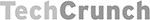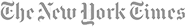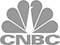### Find Online Tutors in Subjects related to Multivariable Calculus.

Get 1-to-1 learning help through online lessons. If you are looking to learn a subject similar to Multivariable Calculus, tap into the nation’s largest community of private tutors. Wyzant helps more students find face to face lessons, in more places than anyone else. Plus, if you're not happy with any new tutor, we'll gladly refund the cost of your first hour. In addition to Multivariable Calculus tutors, Wyzant can also help you find:

Get the Multivariable Calculus help you need, right when you need it with the convenience of online lessons.